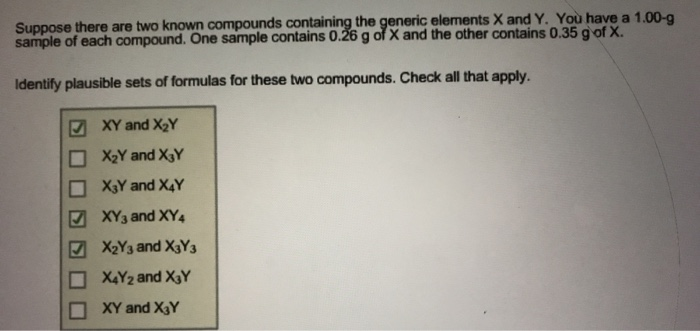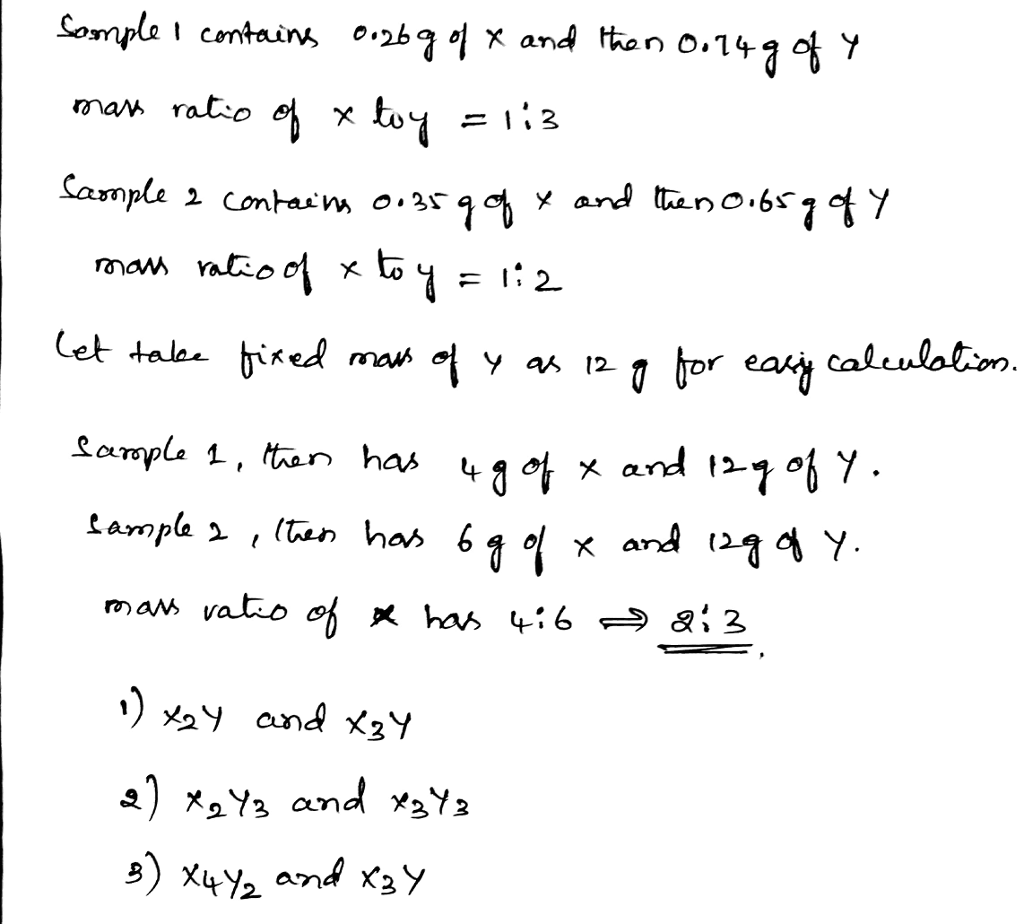# Homework Solution: Suppose there are two known compounds containing the generic elements X and Y. You have a…Suppose there are two known compounds containing the generic elements X and Y. You have a 1.00-g sample of each compound. One sample contains 0.26 g of X and the other contains 0.35 g of X. Identify plausible sets of formulas for these two compounds. Check all that apply. XY and X_2Y X_2Y and X_3Y X_3Y and X_4Y XY_3 and XY_4 X_2Y_3 and X_3Y_3 X_4Y_2 and X_3Y XY and X_3Y